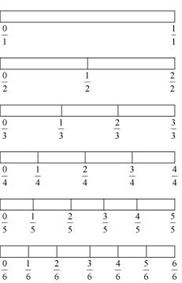# Important Questions for Class 6 Maths Fractions

In this page we have Important Questions for Class 6 Maths Fractions . Hope you like them and do not forget to like , social share and comment at the end of the page.
Question 1
Find the value of the Missing numbers
a) $\frac {2}{3} = \frac {---}{27}$
b) $\frac {2}{---} = \frac {4}{12}$
c) $\frac {1}{---} = \frac {5}{10}$
d) $\frac {7}{8} = \frac {35}{---}$

Question 2
X had 20 chocolates, Y had 50 chocolates and Z had 80 chocolates After 15 days, X eaten up 10 chocolates, Y eaten up 25 chocolates and Z used up 40 chocolates. What fraction did each eat up? Check if each has eaten up an equal fraction of her/his chocolates?

Question 3
Look at the figures and Give true and false about the statements(a) $\frac {1}{2} > \frac {1}{3}$
(b) $\frac {4}{5} > \frac {5}{6}$
c) $\frac {2}{6} < \frac {1}{3}$
d) $\frac {2}{3} > \frac {5}{6}$
e) $\frac {1}{6} > \frac {1}{5}$
f) $\frac {3}{5} > \frac {2}{4}$

Question 4
The following fractions represent just three different numbers. Separate them into three groups of equivalent fractions, by changing each one to its simplest form.
(a) 1/6
(b) 4/20
(c) 3/9
(d) 9/27
(e) 20/120
(f) 11/55
(g) 14/70
(h) 16/96
(i) 33/99
(j) 15/75

Question 5
Find the following
a)$\frac {1}{9} + \frac {2}{9}$
b)$\frac {21}{22} -\frac {10}{22}$
c) $\frac {1}{36} + \frac {17}{36}$
d) $\frac {18}{77} - \frac {11}{77}$
e) $1 - \frac {2}{3}$
f) $1 - \frac {1}{3}$
h) $\frac {2}{3} + \frac {1}{3}$

Question 6
Find the following
a)$\frac {1}{6} + \frac {2}{7}$
b)$\frac {1}{2} - \frac {1}{3}$
c) $\frac {1}{5} + \frac {17}{15}$
d) $\frac {18}{11} - \frac {1}{2}$
e) $\frac {1}{5} + \frac {2}{3}$
f) $\frac {1}{5} - \frac {1}{6}$
g) $\frac {1}{2} + \frac {1}{3} + \frac {1}{4} + \frac {1}{5}$
h) $\frac {1}{4}+ \frac {1}{6} + \frac {1}{8}$

Question 7
Reduce the fraction to simplest form
a)$\frac {20}{25}$
b) $\frac {11}{121}$
c) $\frac {48}{72}$
d) $\frac {125}{625}$
e) $\frac {32}{256}$
f)$\frac {63}{84}$
g) 19/ 38

Question 8
Sonu ate 1/3 part of an apple and the remaining apple was eaten by her brother Monu. How much part of the apple did Monu eat? Who had the larger share? By how much?
Question 9
X finished coloring a picture in 7/12 hour. Y finished coloring the same picture in ¾ hour. Who worked longer? By what fraction was it longer?### Practice Question

Question 1 What is $\frac {1}{2} + \frac {3}{4}$ ?
A)$\frac {5}{4}$
B)$\frac {1}{4}$
C)$1$
D)$\frac {4}{5}$
Question 2 Pinhole camera produces an ?
A)An erect and small image
B)an Inverted and small image
C)An inverted and enlarged image
D)None of the above